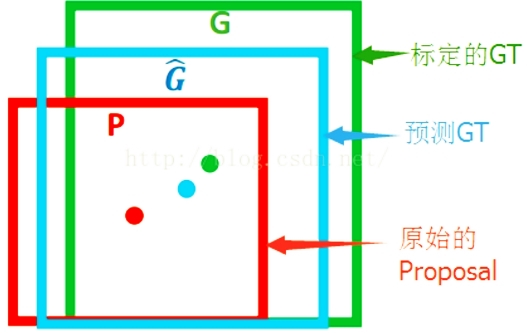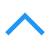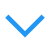# Bounding-box 回归

R-CNN系列均训练了Bounding-box回归器来对窗口进行校正,其目标是学习一种转换关系将预测得到的窗口P映射为真实窗口G(Ground truth).## 变换方式

$\begin{cases} \widehat{G_x}=P_wd_x(P)+P_x \\ \widehat{G_y}=P_hd_y(P)+P_y \end{cases} \tag{仿射}$

$\begin{cases} \widehat{G_w}=P_we^{d_w(P)} \\ \widehat{G_h}=P_he^{d_h(P)} \end{cases}\tag{尺度缩放}$

$\begin{cases} t_x = (G_x− P_x)/P_w \\ t_y = (G_y− P_y)/P_h \\ t_w = \log(G_w/P_w) \\ t_h = \log(G_h/P_h) \end{cases}$

$w_*= \text{argmin}_{\hat w_*}\sum_i^N(t^i_*-s^i_*)^2+\lambda\|\hat w_*\|^2$

### 加旋转角度

$\begin{cases} t_x = (G_x− P_x)/(P_w\cosθ+P_h\sin|θ|) \\ t_y = (G_y− P_y)/(P_w\sin|θ|+P_h\cosθ) \\ t_w = \log(G_w/P_w) \\ t_h = \log(G_h/P_h) \\ ta= (G_a−P_a)/(λ180) \end{cases}$

λ是个常数(λ = 0.5)。

$\begin{cases} t_x = (\cosα(G_x− P_x) + \sinα(G_y− P_y))/P_w \\ t_y = (−\sinα(G_x− P_x) + \cosα(G_y− P_y))/P_h \end{cases}$

scale-invariant translation (SIT)的示例图：posted @ 2018-03-15 11:49  康行天下  阅读(937)  评论(0编辑  收藏